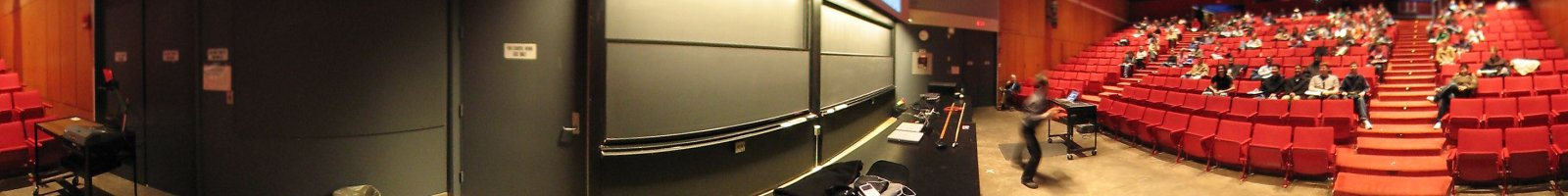## Some teaching notes

 Math 1a, spring 2021 (PDF all lectures) Combined: Math 21a, Fall 2019 (all units) , Units: 1, 2, 3a, 3b, 3c, 4a, 4b, 5, 6, 7, 8, 9, 10, 11, 12, 13, 14, 15, 16, 17. 18. 19. 20. 21. 22. 23. 24. 25. 26. 27. 28. 29. 30. 31. 32. In class exercises: 1, 2, 3, 4, 5, 6, 7, 8, 9, 10, 11, 12, 13, 14, 15, 16, 17, 18, 19, 20, 21, 22, 23, 24, 25, 26, 27, 28, 29, 30, 31, 32,

## Linear algebra and differential equations

• Math 22b (Spring 2019)
• Math21b (Spring 2018)
• Math21b (Spring 2017)
• Math21b (Spring 2016)
• Math21b (Spring 2015)
• Math21b (Fall 2010)
• Math21b (Spring 2010)
• Math21b (Spring 2009)
• Math21b (Spring 2008)
• Math21b (Spring 2007)
• Math21b (Fall 2004)
• Math21b (Fall 2003)

## Probability theory

Oliver Knill, Department of Mathematics, Harvard University, One Oxford Street, Cambridge, MA 02138, USA. SciCenter 432 Tel: (617) 495 5549, Email: knill@math.harvard.edu Quantum calculus blog, Twitter, Youtube, TikTok, Vimeo, Linkedin, Scholar Harvard, Academia, Harvard Academia, Google Scholar, ResearchGate, Slashdot, Ello, Webcam, 2021: send email for appointment